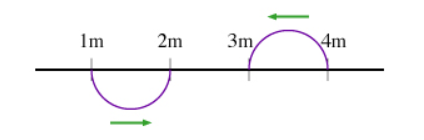# Problem: First, consider two wave pulses on a string, approaching each other. (Figure 1) Assume that each moves with speed 1 meter per second. The figure shows the string at time  t = 0. The effect of each wave pulse on the string (which is the medium for these wave pulses) is to displace it up or down. The pulses have the same shape, except for their orientation. Assume that each pulse displaces the string a maximum 0.5 meters, and that the scale on the x axis is in meters. Part A At time t= 1s, what will be the displacement Δy at point x = 2.5 m? The same process of superposition is at work when we talk about continuous waves instead of wave pulses. Consider a sinusoidal wave as in the figure. Part BHow far Δx to the left would the original sinusoidal wave to be shifted to give a wave that would completely cancel the original? The variable λ in the picture denotes the wavelength of the wave.

###### FREE Expert Solution

Part A

From the figure, at x = 2.5 m, the crest of one wave will fall on the trough of another wave.

82% (191 ratings)###### Problem Details

First, consider two wave pulses on a string, approaching each other. (Figure 1) Assume that each moves with speed 1 meter per second. The figure shows the string at time  t = 0. The effect of each wave pulse on the string (which is the medium for these wave pulses) is to displace it up or down. The pulses have the same shape, except for their orientation. Assume that each pulse displaces the string a maximum 0.5 meters, and that the scale on the x axis is in meters.Part A

At time t= 1s, what will be the displacement Δy at point x = 2.5 m?

The same process of superposition is at work when we talk about continuous waves instead of wave pulses. Consider a sinusoidal wave as in the figure.

Part B

How far Δx to the left would the original sinusoidal wave to be shifted to give a wave that would completely cancel the original?

The variable λ in the picture denotes the wavelength of the wave.

Frequently Asked Questions

What scientific concept do you need to know in order to solve this problem?

Our tutors have indicated that to solve this problem you will need to apply the Waves on a String concept. You can view video lessons to learn Waves on a String. Or if you need more Waves on a String practice, you can also practice Waves on a String practice problems.

What professor is this problem relevant for?

Based on our data, we think this problem is relevant for Professor Liao's class at IU.